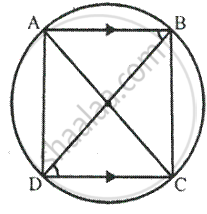Share

# In a Cyclic-trapezium, the Non-parallel Sides Are Equal and the Diagonals Are Also Equal. Prove It. - Mathematics

Course

#### Question

In a cyclic-trapezium, the non-parallel sides are equal and the diagonals are also equal. Prove it.

#### SolutionA cyclic trapezium ABCD in which AB ∥ DC and AC and BD are joined.
To prove-
(ii) AC = BD
Proof :
∵ chord AD subtends ∠ABD and chord BC subtends BDC
At the circumference of the circle.

But ∠ABD = ∠BDC   [proved]

Now in ∆ADC and ∆ BCD

DC = DC      [Common]

∠CAD = ∠CBD           [angles in the same segment]

By Side – Angle – Side criterion of congruence, we have

∴ ∆ADC ≅ ∆BCD       [ SAS axion]

The corresponding parts of the congruent triangle are congruent

∴ AC = BD       [c.p.c.t]

Is there an error in this question or solution?